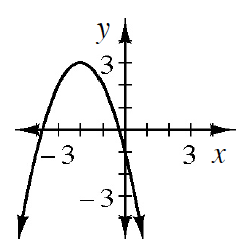### Home > INT2 > Chapter 11 > Lesson 11.2.1 > Problem11-60

11-60.

Wendell also has a water balloon to launch, but he gets turned around and launches his balloon in the wrong direction! The path of his balloon is modeled by the function $y = −(x + 2)^2 + 3$ where $x$ is the horizontal distance in yards in front of the goal line and y is the height of the balloon in yards.

1. Make a complete graph of the function.2. What is the domain of the function? What domain makes sense for this context?

The domain is all real numbers.
For this context, a reasonable domain would be $-3.7 ≤ x ≤ -0.3$.

3. What is the maximum value of the function? What does this tell you about the path of the balloon?

The maximum value is the greatest $y$-value.

4. Use your answers from parts (a) through (c) to completely describe the path of the balloon.

The $x$ intercepts are the two places where the balloon is launched and where it lands.